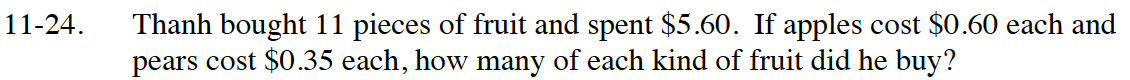### Home > CC4 > Chapter 11 > Lesson 11.1.2 > Problem11-24

11-24.

Thanh bought 11 pieces of fruit and spent $5.60. If apples cost$0.60 each and pears cost \$0.35 each, how many of each kind of fruit did he buy? Homework Help ✎Let x = the number of apples he bought
Let y = the number of pears he bought
Write a system of equations using the given information.

0.60x + 0.35y = 5.60
x + y = 11

Solve the system of equations for x and using either the Substitution Method or the Elimination Method.